Next: An efficient algorithm for Up: Algorithm for minimizing the Previous: A quasi-Newton method for

# The limited memory BFGS method

Nocedal (1980) derives a technique that partially solves the storage problem caused by the BFGS update. Instead of keeping all theand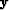from the past iterations, we update the Hessian using the information from the l previous iterations, where l is given by the end-user. This implies that when the number of iterations is smaller than l, we have the usual BFGS update, and when it is larger than l, we have a limited memory BFGS (L-BFGS) update.

I give the updating formulas of the Hessian as presented by Nocedal (1980). First, we define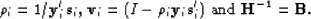As described above, when k, the iteration number, obeys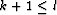, where l is the storage limit, we have the BFGS update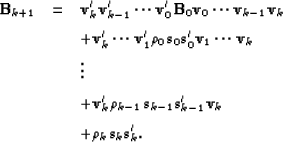(75)
For k+1 > l we have the limited memory update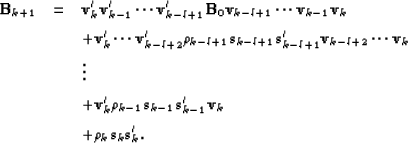(76)
These equations show how the update of the Hessian is calculated.

Usually the L-BFGS method is implemented with a line search for the step length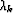to ensure a sufficient decrease of the misfit function. Convergence properties of the L-BFGS method are guaranteed ifin equation () satisfies the Wolfe conditions Kelley (1999):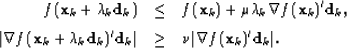(77) (78)andare constants to be chosen a priori and. Forandwe set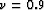and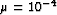as proposed by Liu and Nocedal (1989). Equation () is a sufficient decrease condition that all line search algorithms must satisfy. Equation () is a curvature condition. The line search algorithm has to be carefully designed since it absorbs most of the computing time. I programmed a line search based on the More and Thuente (1994) method. Because the line search is time consuming, the step length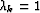is always tested first. This procedure saves a lot of computing time and is also recommended by Liu and Nocedal (1989). I now give the algorithm used to minimize any objective function involving nonlinear problems.Next: An efficient algorithm for Up: Algorithm for minimizing the Previous: A quasi-Newton method for
Stanford Exploration Project
5/5/2005